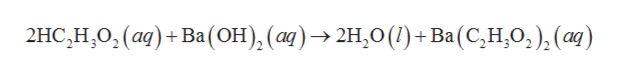# Consider the unbalanced equation for the neutralization of acetic acid:αHC2H3O2(aq)+βBa(OH)2(aq) →γH2O(l)+δBa(C2H3O2)2(aq)How would this equation looked balanced

Question
32 views

Consider the unbalanced equation for the neutralization of acetic acid:

αHC2H3O2(aq)+βBa(OH)2(aq) →γH2O(l)+δBa(C2H3O2)2(aq)

How would this equation looked balanced

check_circle

Step 1

The unbalanced chemical equation is given by as follows:

Step 2

The chemical equation is balanced by using the law of conservation of mass in which both reactant and product contain the same number of atoms. The equation is balanced by multiplying the required coefficient number.

Step 3

In the given chemical equation, put 1 in place of beta and delta, and put 2 in place of alpha a...help_outlineImage Transcriptionclose2HC, Н, О, (а) + Ва(ОН), (а) > 2Н,0 () + Ba (С, Н,О,), (ӕ) fullscreen

### Want to see the full answer?

See Solution

#### Want to see this answer and more?

Solutions are written by subject experts who are available 24/7. Questions are typically answered within 1 hour.*

See Solution
*Response times may vary by subject and question.
Tagged in

### General Chemistry